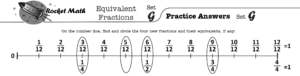## Facts practice: does it belong in middle school math?

It sure does, if you’re seeing this happen in your class!

Most middle school math teachers confide to me that their classrooms are negatively impacted by the number of students who stop to count out facts on their fingers.  Their issue was always what to do during facts practice with the other students who do know their facts.  It has taken a couple of years but I have put together a package of pre-algebra skills that are worth middle school students’ time practicing which are available in the Universal Subscription. Because the routine of Rocket Math is the same whether the students are practicing basic multiplication facts or learning equivalent fractions you’ll be able to manage all these different levels during the same ten-minute session.

Teachers know it is imperative that finger-counting middle schoolers get practice learning their facts.  Rocket Math is an excellent way to do that.  They will develop fluency and automaticity with the basic facts in an operation in a semester and from then on your lessons will be much easier.  Not only that, but a much higher proportion of the students will be finishing assignments.  There is a “Placement Probe” that can identify students who know their facts in about one minute. The students who know the basic facts of multiplication and division can be placed into the pre-algebra practice programs.

. Students probably ought to begin with the Factors program. What are the factors of 24? Answer: 1 and 24, 2 and 12, 3 and 8, 4 and 6. This is what students learn by memory from doing this program. Students practice with a partner, take a daily one minute timing, fill in a Rocket Chart, just like regular Rocket Math. Students learn all the factors for these numbers in this sequence: 12, 36, 24, 48, 18, 32, 16, 64, 10, 40, 20, 72, 8, 25, 50, 6, 21, 30, 60, 15, 45, and 100.EQUIVALENT FRACTIONS.  Students need to know that six-eighths is equivalent to three-fourths and that four-twelfths is equivalent to one-third.  While they can calculate these, it is very helpful to know the most common equivalent fractions by memory.  One of the most common problems students have in fractions is not “reducing their answers to simplest form.”  Equivalent fractions will help students commit 100 common equivalent fractions to memory.  Each set (A through Z) has four fractions which are displayed on a fraction number line.  Students frequently learn fractions equivalent to one,such as ten-tenths, as well as fractions that can’t be reduced, for example three-fourths is equivalent to three-fourths.  Using the fraction number line will help with student understanding of why those fractions are equivalent.

(Adding and subtracting positive and negative numbers).  Integers displays problems on a vertical number line and then teaches students two rules about how to solve problems that add or subtract positive and negative numbers.

Rule 1: Go up when you add a positive number OR subtract a negative number.
Rule 2: Go down when you subtract a positive number OR add a negative number.

Students gradually learn several variations of all four types of problems.  They practice with the number line on each page and then have a chance to build fluency on the top half of the page as they work with their partner.  You will probably not be surprised that there is a one-minute test on each set.  The goals are slightly different than before.  Students are to be 100% accurate and to complete at least 80% of their rate at answering simple addition and subtraction problems.

10s, 11s, 12s Multiplication and 10s, 11s, 12s Division facts are also available in the Universal Subscription.  If you have students who think they know the basic facts, but need review, putting them into either of these programs will review the 1s through 9s facts, teach them new ones and allow them to save face.

Among these five programs there are good things for ALL middle school math students to learn, even the more advanced students.  This will enable a math teacher to devote ten minutes a day to fact practice without holding anyone back.  Everyone will have something meaningful to practice during that time.  I think this could be a huge step forward for a lot of middle school MATH classrooms.